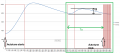# Calculating Kp,Ki & kd values with Astrom-Hagglund(Relay) Method

#### Vindhyachal Takniki

Joined Nov 3, 2014
519
Hi,

I was reading your blog post here: http://brettbeauregard.com/blog/2012/01/arduino-pid-autotune-library/ and trying to implement autotune library to maintain temperature in industrial ovens.

1. We bought a Delta Electronics PID and oven and see how it Delta Electronics PID controller do autotune. Below is the graph we obtained
2. Since it looks like Astrom-Hagglund(Relay) Method, sowe tried to get Kc & Tu values from it.
4. a) Tu is calculated in seconds
b) Kc = (4*D)/(pi*A)
D=100 taken since relay is 100% on or off only
A=Difference between +peak and -peak value.

5. One important thing we note that its not symmetrical oscillation, (zero to +peak) is higher than (zero to -peak) value.

6. But when we put it in formula as in link above menetioned code, outout is always saturated high. I think we have to normailize outut formula before using, but dont know how to do it or anything we are doing in our code.

Code:
const float32_t c_output_max = 100.0f;
const float32_t c_output_min = 0.0f;

float32_t input_temperature;
float32_t setpoint_temperature;
float32_t output_duty_cycle;

float32_t i_term;
float32_t last_input;

//ku = 12.60633212609071966486208026713f;   units: per degree celsius
//tu = 820  seconds
float32_t kp = 7.563799f;   //kp = 0.6*Ku
float32_t ki = 0.018448f;  // ki = 1.2*Ku/Tu
float32_t kd = 775.289425f;    // ki = 0.075*Ku*Tu

float32_t sample_time = 1.0f;  // 1 second

//sample_time + derivative_kick + On-The-Fly Tuning Changes + integral windup +
void compute_pid_duty_cycle(void)
{
float32_t error;
float32_t d_input;

/* get error */
error = setpoint_temperature - input_temperature;

/* integral error */
i_term = i_term + (error * ki);
if(i_term > c_output_max)
{
i_term = c_output_max;
}
else if(i_term < c_output_min)
{
i_term = c_output_min;
}
else
{
}

/* differential error */
d_input = input_temperature - last_input;

/*Compute PID Output*/
output_duty_cycle = (kp * error) + (i_term) - (kd * d_input);
if(output_duty_cycle > c_output_max)
{
output_duty_cycle = c_output_max;
}
else if(output_duty_cycle < c_output_min)
{
output_duty_cycle = c_output_min;
}
else
{
}

/*Remember some variables for next time*/
last_input = input_temperature;

} /* function ends here */#### Vindhyachal Takniki

Joined Nov 3, 2014
519
Have written below code, but outout is very fluctating and then clamped to either zero or highest.

This is general three gains Kp, Ki and Kd.
I think one reason might be: dimensions dont match on both sides . Though i am not sure of this.

1. kp has unit : (change in output)/celsius.
since Kp is proportional to D/A , where D= change in output which we take 0 to 100, since its relay method.
A= change in temperature.

2. ki = 1.2*Ku/Tu so its units: (change in output)/(celsius * second).

3. ki = 0.075*Ku*Tu so its units: (change in output * second)/(celsius).

4.While on left of equation , which is outout it is dimension less or percentage

I have checked another PID controller from another company they also used porportional band, ; https://web-material3.yokogawa.com/IM05P01C31-01EN.pdf

But i dont get how to caculate it.
Defination sats: " It is amount of change in input (or deviation), as a percent of span, required to cause the control output to change from 0% to 100% "
For example in our test, with set point 70C, max goes to 78.6, minimum goes to 69.0,

sensor span is -200C to + 600C

How to calculate it?

is it : (78.6-69.0)*100/(600+200C) ??

Code:
void compute_pid_duty_cycle(void)
{
float32_t error;
float32_t temp_integral;
float32_t derivative;

/* get error */
error = setpoint_temperature - input_temperature;

if(first_time)
{
first_time = 0U;
previous_err = error;
}

/* integral error */
integral = integral + error;   //dt = 1sec
temp_integral = integral * ki;

if(temp_integral > c_output_max)  //integral windup up
{
temp_integral = c_output_max;
}
else if(temp_integral < c_output_min)
{
temp_integral = c_output_min;
}
else
{
}

/* differential error */
derivative = error - previous_err;     //dt = 1seconds

/* Compute PID Output */
output_duty_cycle = (kp * error) + (temp_integral) + (kd * derivative);
if(output_duty_cycle > c_output_max)
{
output_duty_cycle = c_output_max;
}
else if(output_duty_cycle < c_output_min)
{
output_duty_cycle = c_output_min;
}
else
{
}

/* Remember some variables for next time */
previous_err = error;

} /* function ends here */

#### tsan

Joined Sep 6, 2014
116
1. We bought a Delta Electronics PID and oven and see how it Delta Electronics PID controller do autotune. Below is the graph we obtained
2. Since it looks like Astrom-Hagglund(Relay) Method, sowe tried to get Kc & Tu values from it.
I think it is some step response method instead of relay method. There is no sustained oscillation and temperature should fluctuate around original setpoint. On the test, actual temperature increases higher like in step response test.

Have written below code, but outout is very fluctating and then clamped to either zero or highest.
It would be good to start with a P-controller only to see that the basic functionality is correct. Then add I-part and D-part.
Defination sats: " It is amount of change in input (or deviation), as a percent of span, required to cause the control output to change from 0% to 100% "
For example in our test, with set point 70C, max goes to 78.6, minimum goes to 69.0,

sensor span is -200C to + 600C

How to calculate it?

is it : (78.6-69.0)*100/(600+200C) ??
I think, that error should be calculated in percents. Controller output is also defined so, that 100% is nominal (could correspond to maximum power of the heater). If we define, that 100C temperature is 100%, then we can calculate an example based on the given definition. Example case:
-set point 70C
-actual temperature 60C
->error (70-60)/100=10%
If the gain of P-controller is 10, controller output is 10%*10 = 100%.